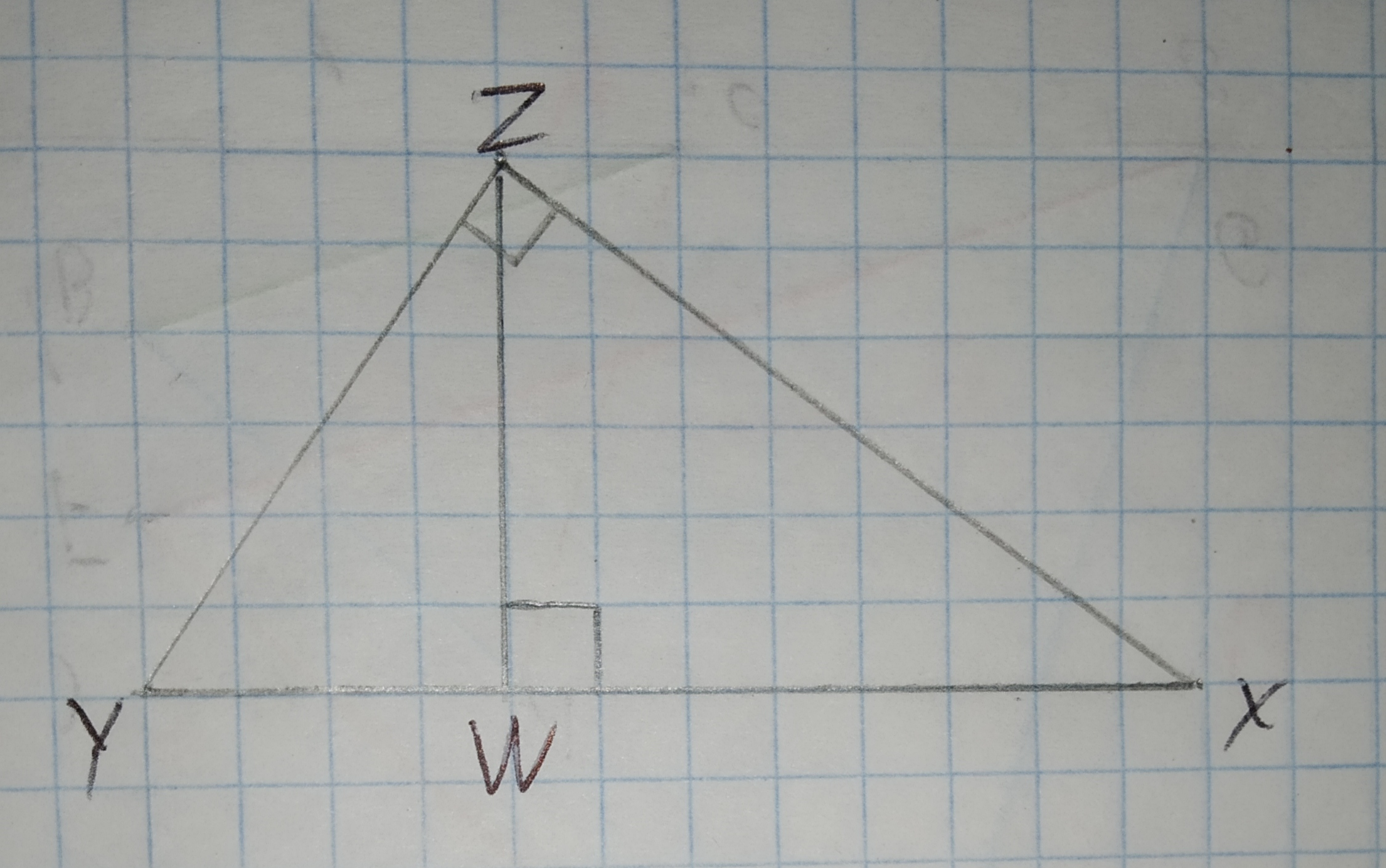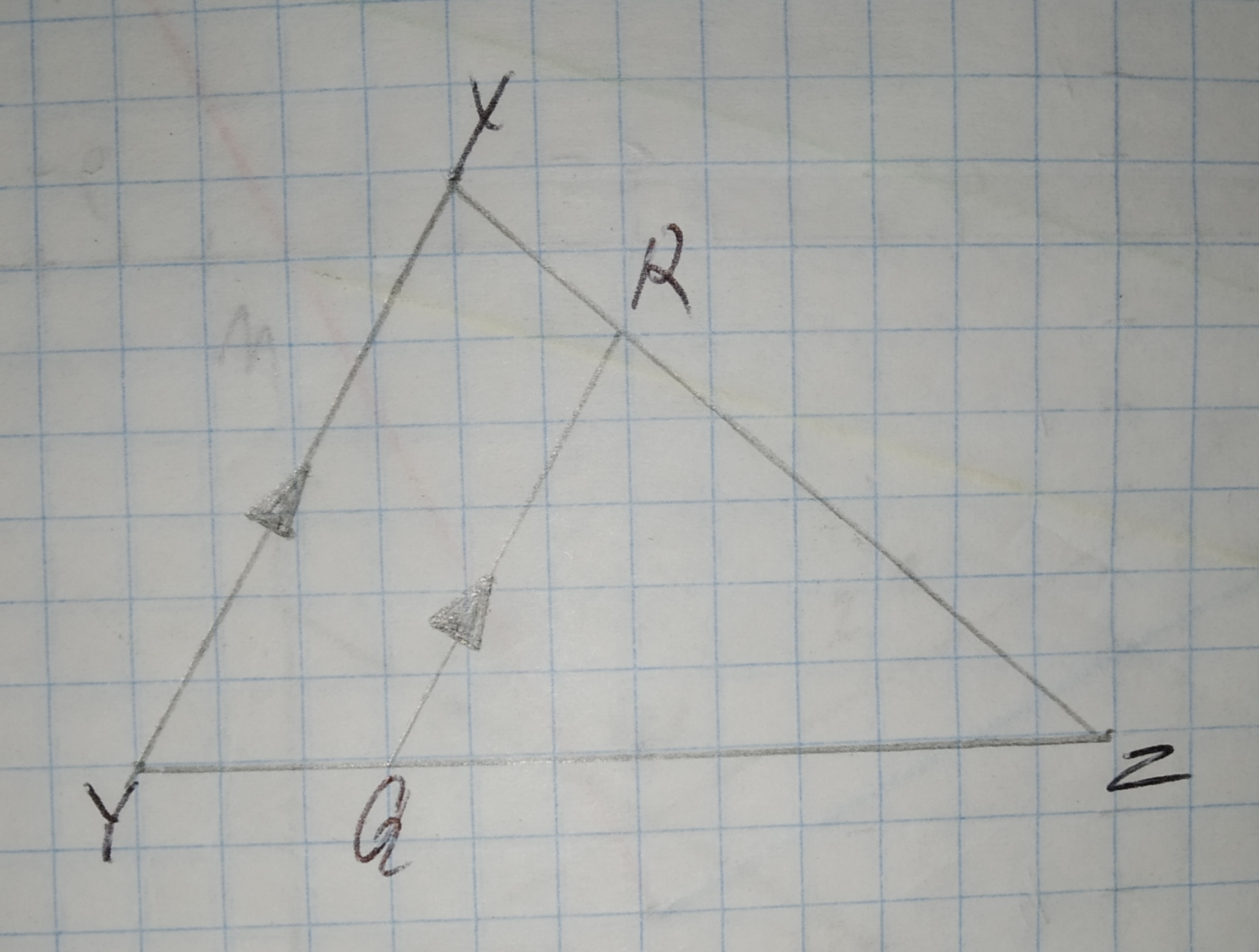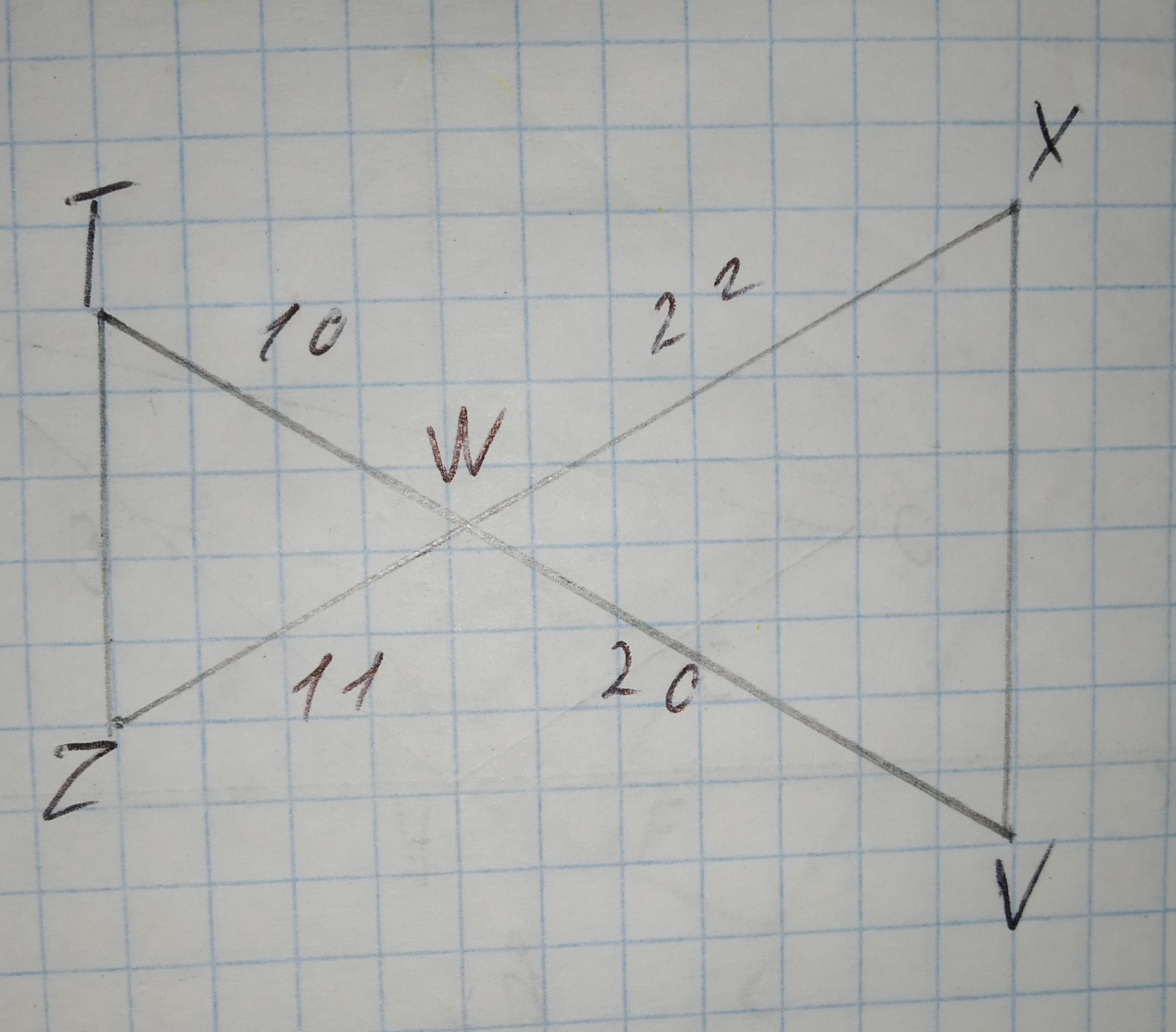# Compute the similarity dimension of a strictly self-similar fractal with a replacement ratio of 5 and a scaling ratio of 4. Round to the nearest thousandth.Question
SimilarityCompute the similarity dimension of a strictly self-similar fractal with a replacement ratio of 5 and a scaling ratio of 4. Round to the nearest thousandth.2021-03-08
Formula used:
The similarity dimension of a strictly-similar fractal is given by
$$\displaystyle{D}=\frac{{{\log{{N}}}}}{{{\log{{r}}}}}$$
Where N is the replacement ratio of the fractal and ris the scaling ratio.
Calculation:
We have, N = 5 and r = 4. Now, use the formula for similarity dimension D of square fractal to calculate it as shown below.
$$\displaystyle{D}=\frac{{{\log{{5}}}}}{{{\log{{4}}}}}={1.161}$$

### Relevant QuestionsWhat is the ratio of the surface areas of similar solids whose similarity ratio is 4 : 7?Find the similarity ratio of two triangles with areas $$\displaystyle{81}{f}{e}{e}{t}^{{2}}{\quad\text{and}\quad}{100}{f}{e}{e}{t}^{{2}}$$.Find the similarity ratio of two circles with areas $$\displaystyle{75}\pi{c}{m}^{{2}}{\quad\text{and}\quad}{27}\pi{c}{m}^{{2}}$$.Given $$\displaystyle\triangle{D}{E}{F}{\quad\text{and}\quad}\triangle{E}{G}{F}$$ in the diagram below, determine if the triangles are similar. If so, write a similarity statement, and state the criterion used to support your claim.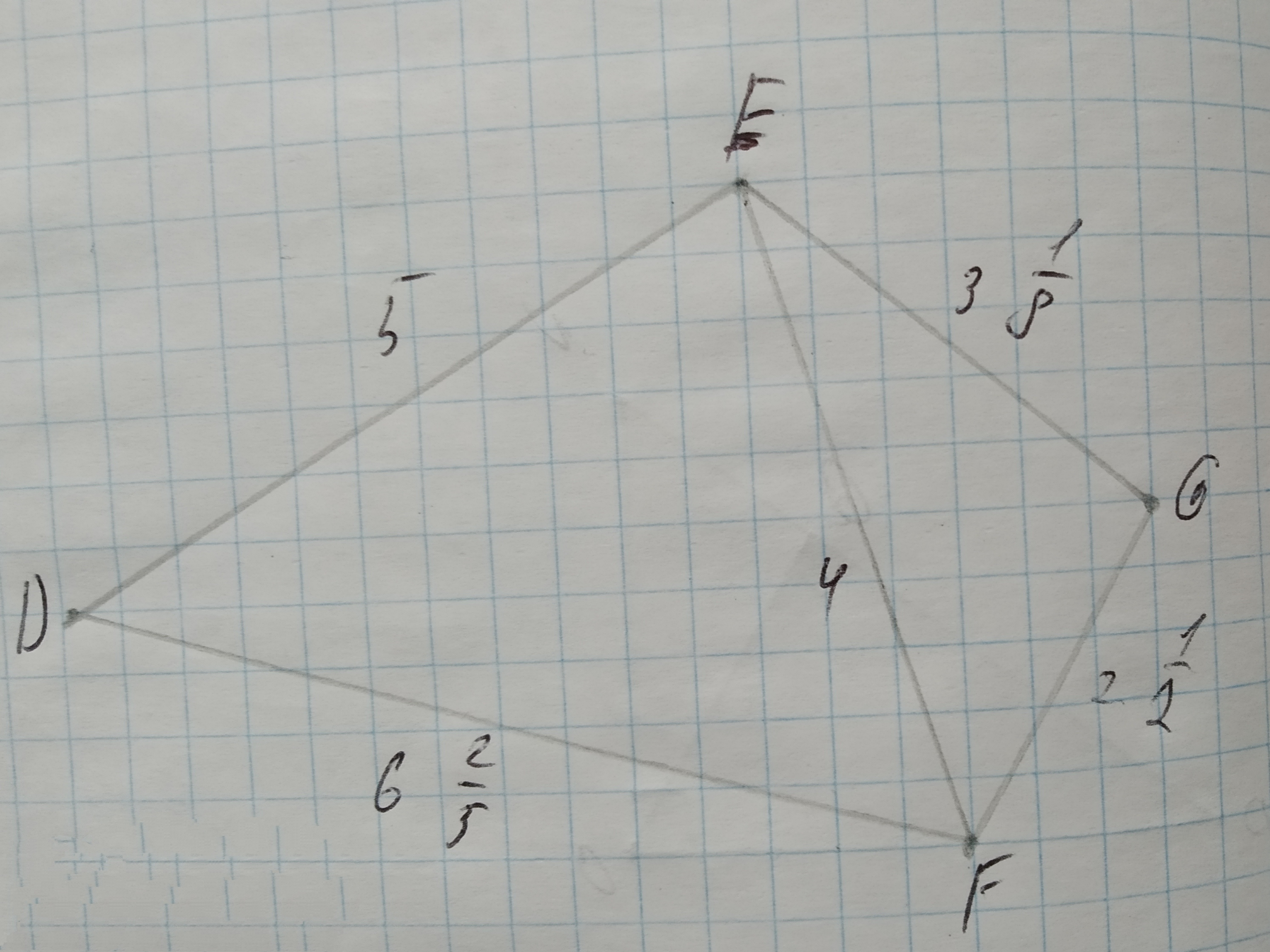To determine:Whether each pair of figures is similar and also write similarity statement and scale factor.
Given information:
Given the figure.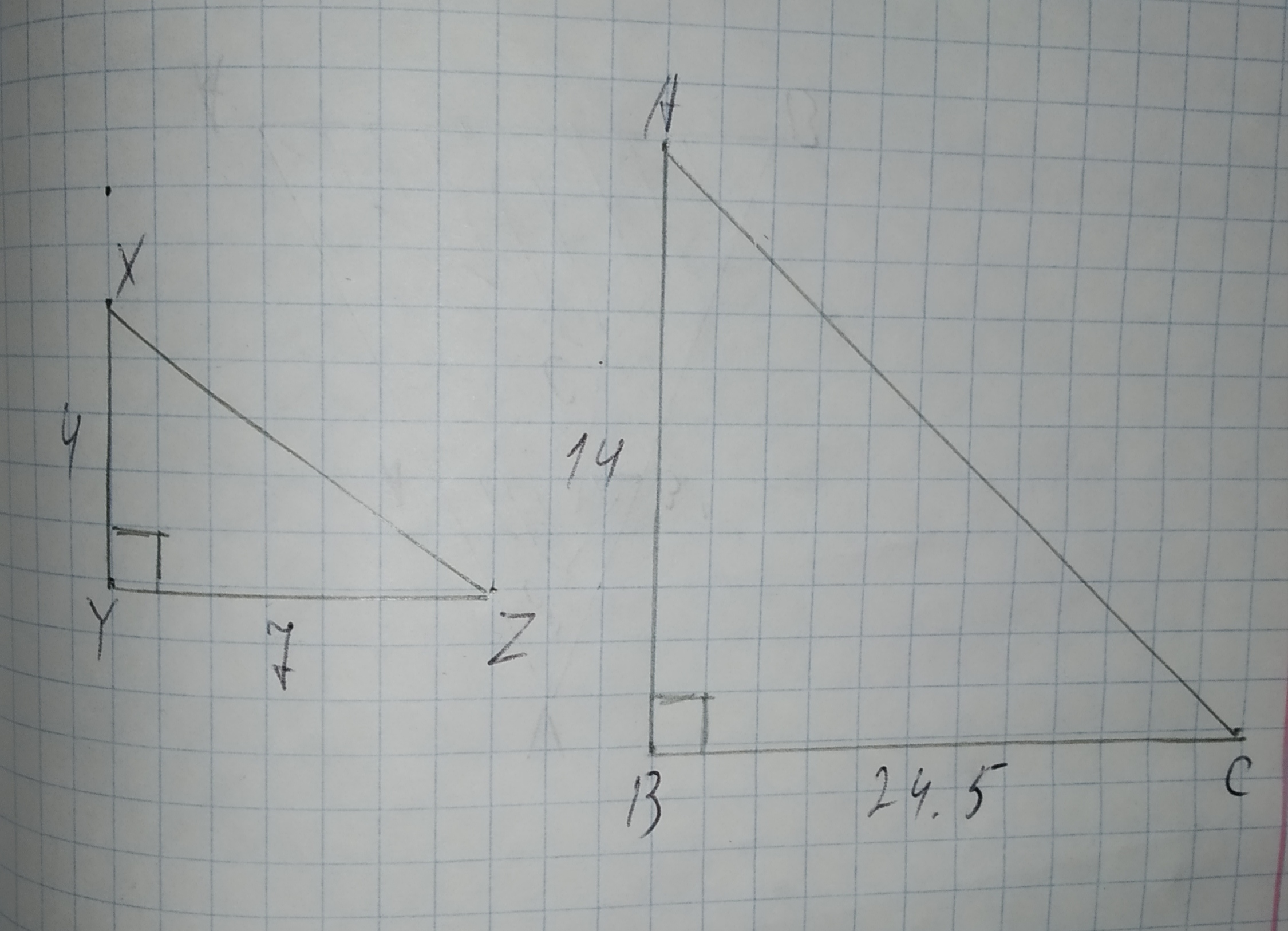Using Similarity Express x in terms of a, b, and c.
Given:
The given pair of triangles is similar.
The given pair of triangle is,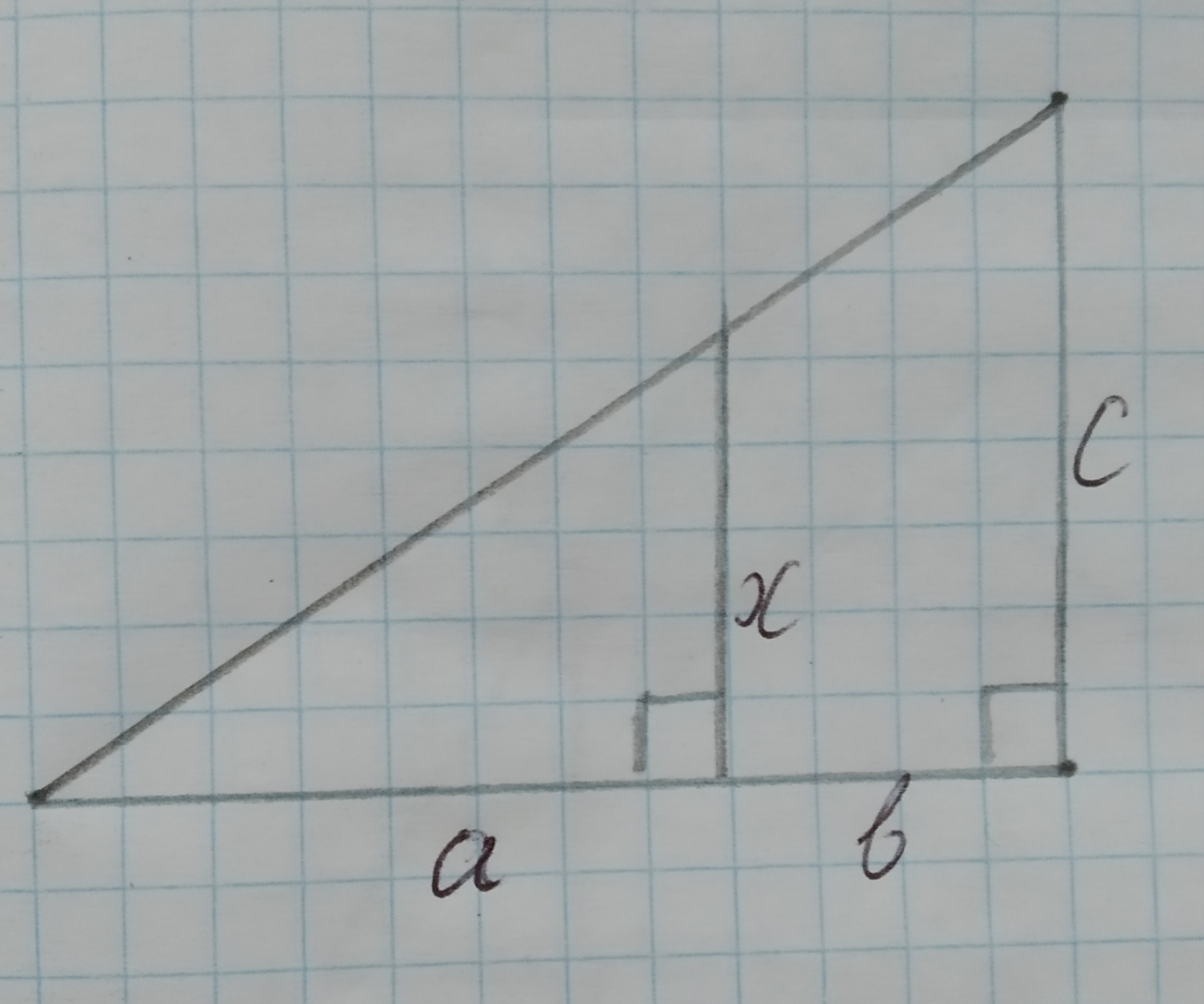Give the explanation for the relation between the scale factor and the similarity ratio.
Given:
The given statement is that the MNOP is a dilation of ABCD.To write three similarity statements about the triangles and the reason that they are similar.
Given that